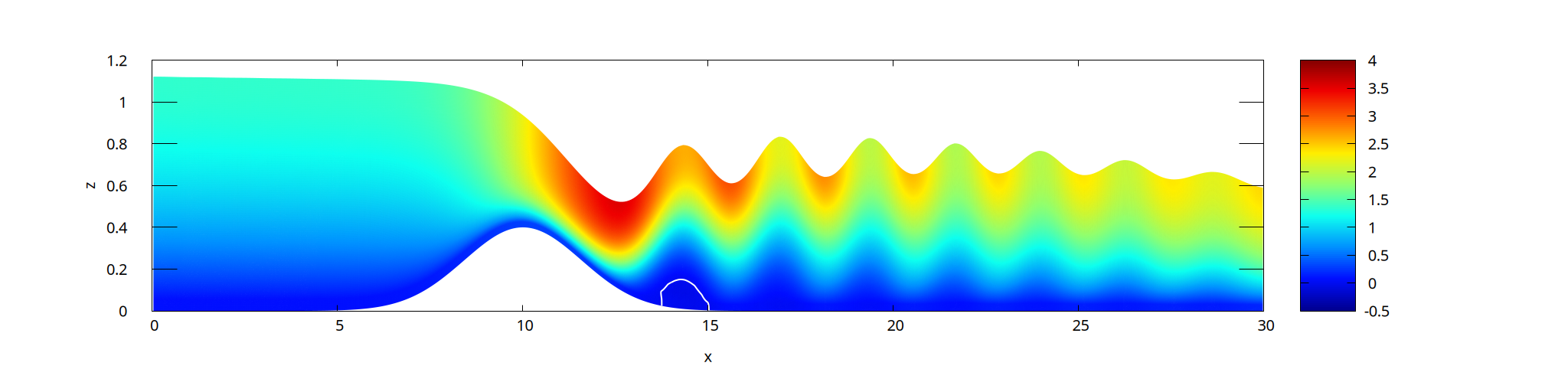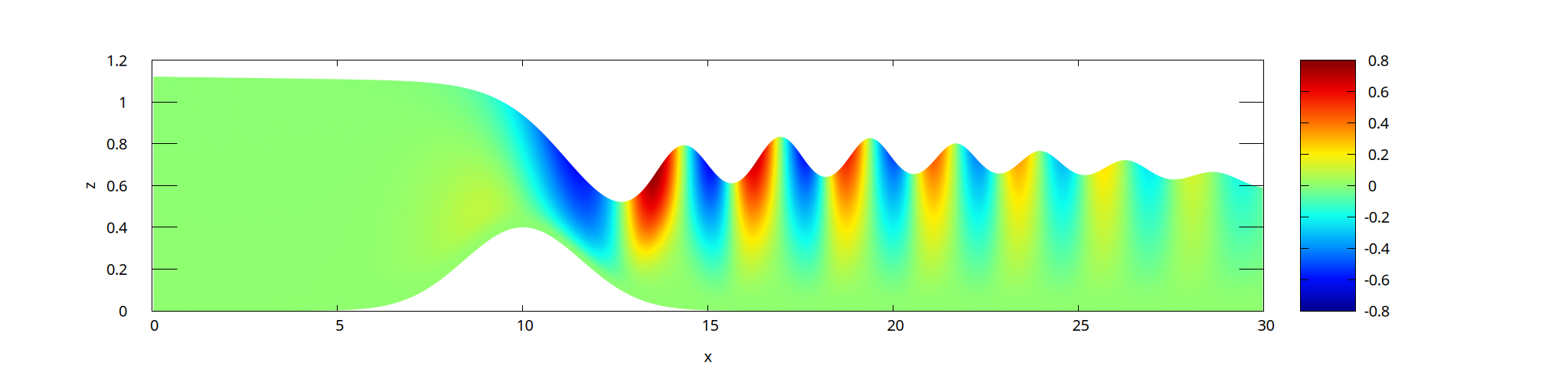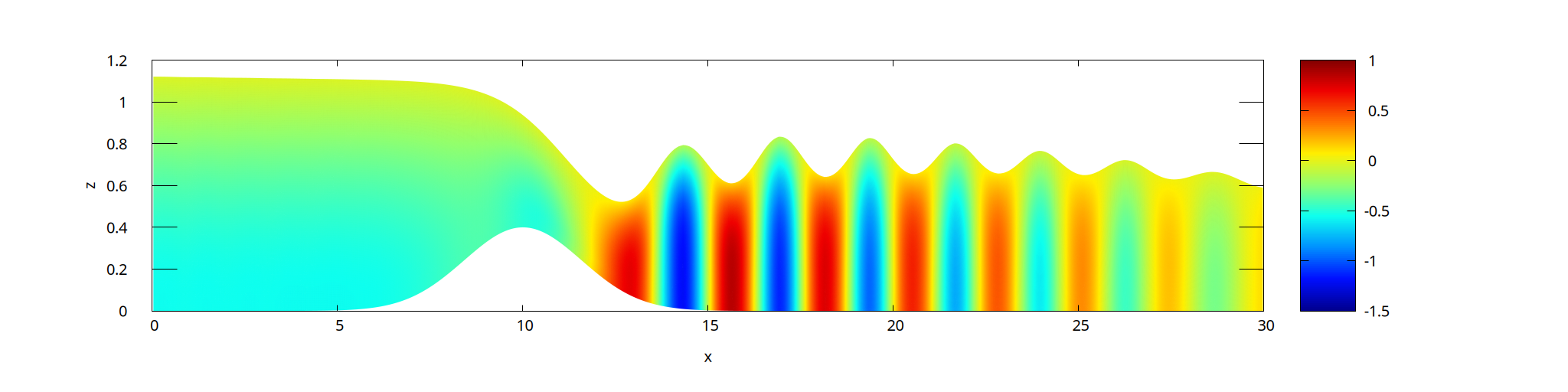# Transcritical flow over a bump with multiple layers

This test case is similar to layered.c but using simpler (and more physical) boundary conditions. Both hydrostatic and non-hydrostatic solutions are obtained. The non-hydrostatic solution is compared with a DNS solution using the Navier-Stokes/VOF solver.

More details are given in Popinet (2020).

#include "grid/multigrid1D.h"
#if !ML
# include "saint-venant.h"
#else // ML
# include "layered/hydro.h"
# define phi q
# if !HYDRO
#   include "layered/nh.h"
# endif
# include "layered/remap.h"
# include "layered/perfs.h"
#endif // ML

The primary parameters are the flow rate, water level, viscosity, bump amplitude and ramping time.

const double QL = 1., HR = 0.6, NU = 1e-2, BA = 0.4, T0 = 10;

int main()
{

The domain is 30 meters long.

  L0 = 30. ;
G = 9.81;
N = 512; // less damping with 1024

The viscosity is set to NU and 20 layers are used to discretise vertically.

  nu = NU;
nl = 20; // going to 30 changes very little
#if ML
#if NOMETRIC
max_slope = 0.;
#endif
#if !HYDRO
NITERMIN = 2;
#endif
#endif
run();
}

## Initialisation and boundary conditions

The inflow is a parabolic profile with a total flow rate Q. The function below computes the height zc of the middle of the layer and returns the corresponding velocity.

#if !ML
double uleft (Point point, scalar s, double Q)
{
double zc = zb[];
for (int l = 0; l < s.l; l++)
zc += layer[l]*h[];
zc += layer[s.l]*h[]/2.;
return 3./2.*Q/h[]*(1. - sq(zc/h[] - 1.));
}
#else

For the multilayer solver, we need some trickery to define the inflow velocity profile as a function of z. We need to sum the layer thicknesses to get the total depth H and the height zc of the current layer (of index point.l).

double uleft (Point point, scalar s, double Q)
{
double H = 0.;
double zc = zb[];
for (int l = - point.l; l < nl - point.l; l++) {
H += h[0,0,l];
if (l < 0)
zc += h[0,0,l];
}
zc += h[]/2.;
return 3./2.*Q/H*(1. - sq(zc/H - 1.));
}
#endif

We initialise the topography and the initial thickness of each layer h.

event init (i = 0)
{
double a = 5.;
foreach() {
zb[] = BA*exp(- sq(x - 10.)/a);
#if !ML
h[] = HR - zb[];
#else
foreach_layer()
h[] = (HR - zb[])/nl;
#endif
}

The height of the free-surface \eta is imposed on the right boundary.

  eta[right] = dirichlet(HR);

Boundary conditions are set for the inflow velocity and the outflow of each layer.

#if !ML
for (vector u in ul) {
u.n[left] = dirichlet (uleft (point, _s, QL*(t < T0 ? t/T0 : 1.)));
u.n[right] = neumann(0.);
}
h[right] = dirichlet(HR);
#else
u.n[left] = dirichlet (uleft (point, _s, QL*(t < T0 ? t/T0 : 1.)));
u.n[right] = neumann(0);
#endif

In the non-hydrostatic case, a boundary condition is required for the non-hydrostatic pressure \phi of each layer.

#if !HYDRO && ML
phi[right] = dirichlet(0.);
#endif
}

We can optionally add horizontal viscosity.

#if 0
event viscous_term (i++)
{
// add horizontal viscosity (small influence)
#if HYDRO
scalar * list = {u.x};
#else
scalar * list = {u.x,w};
#endif
scalar d2u[];
foreach_layer()
for (scalar s in list) {
foreach()
d2u[] = (u.x + u.x[-1] - 2.*u.x[])/sq(Delta);
foreach()
u.x[] += dt*nu*d2u[];
}
}
#endif

We check for convergence.

scalar etac[];

event logfile (t += 0.1; i <= 100000) {
FILE * fp = fopen ("dh", "w");
foreach()
fprintf (fp, "%g %g\n", x, eta[] - etac[]);
fclose (fp);
double dh = change (eta, etac);
if (i > 1 && dh < 5e-5)
return 1;
}

Uncomment this part if you want on-the-fly animation.

#if 0
#include "plot_layers.h"
#endif

## Outputs

We save profiles at regular intervals.

event profiles (t += 5)
{
foreach (serial) {
#if !ML
double H = h[];
#else
double H = 0.;
foreach_layer()
H += h[];
#endif
fprintf (stderr, "%g %g %g\n", x, zb[] + H, zb[]);
}
fprintf (stderr, "\n\n");
}

For the hydrostatic case, we compute a diagnostic vertical velocity field w. Note that this needs to be done within this event because it relies on the fluxes hu and face heights hf, which are only defined temporarily in the multilayer solver.

#if HYDRO
scalar w = {-1};

event update_eta (i++)
{
if (w.i < 0)
w = new scalar[nl];
vertical_velocity (w, hu, hf);

The layer interface values are averaged at the center of each layer.

  foreach() {
double wm = 0.;
foreach_layer() {
double w1 = w[];
w[] = (w1 + wm)/2.;
wm = w1;
}
}
}
#endif // HYDRO

We also save the velocity and non-hydrostatic pressure fields.

event output (t = end)
{
foreach (serial) {
double z = zb[];
#if HYDRO
printf ("%g %g %g %g\n", x, z, u.x[], w[]);
foreach_layer() {
z += h[];
printf ("%g %g %g %g\n", x, z, u.x[], w[]);
}
#elif !ML
vector u = ul;
scalar w = wl;
printf ("%g %g %g %g\n", x, z, u.x[], w[]);
int l = 0;
for (u,w in ul,wl) {
z += layer[l++]*h[];
printf ("%g %g %g %g\n", x, z, u.x[], w[]);
}
#else // ML
printf ("%g %g %g %g %g\n", x, z, u.x[], w[], phi[]);
foreach_layer() {
z += h[];
printf ("%g %g %g %g %g\n", x, z, u.x[], w[], phi[]);
}
#endif // ML
printf ("\n");
}
#if HYDRO
delete ({w});
#endif
printf ("# end = %g\n", t);
}

## Results

We compare the hydrostatic results obtained with the layered solver and those obtained with the Saint-Venant multilayer code.

set yr [0.5:1.2]
set xlabel 'x'
set ylabel 'z'
plot '../gaussian-hydro/log' u 1:2 w l t 'Multilayer', \
'../gaussian-stvt/log' u 1:2 w l t 'De Vita et al.'Evolution of the free surface (hydrostatic). (script)

The results which follow are obtained with the non-hydrostatic solver.

plot 'log' u 1:2 w l t ''Evolution of the free surface (non-hydrostatic) (script)

We compare the results obtained with the layered solver to those obtained with the Navier-Stokes VOF solver (and without metric).

plot 'log' w l t 'Multilayer', \
'../../examples/gaussian-ns/log' w l t 'Navier-Stokes VOF'Evolution of the free surface for both solvers (script)

plot 'log' index 11 w l t 'Multilayer', \
'../../examples/gaussian-ns/log' index 'prof70' w l t 'Navier-Stokes VOF', \
'../gaussian-nometric/log' index 12 w l t 'no metric'Final free surface profiles (script)

set term PNG enhanced font ",15" size 2048,512
set yr [0:1.2]
set output 'vel.png'
set pm3d
set pm3d map interpolate 10,4
unset key
# jet colormap
set palette defined ( 0 0 0 0.5647, 0.125 0 0.05882 1, 0.25 0 0.5647 1,	\
0.375 0.05882 1 0.9333, 0.5 0.5647 1 0.4392, 0.625 1 0.9333 0, 0.75 1 0.4392 0,	\
0.875 0.9333 0 0, 1 0.498 0 0 )
set contour base
set cntrparam levels discrete 0
set cntrlabel onecolor
set cntrparam bspline
splot 'out' u 1:2:3 lt 3 lc rgb "#ffffff" lw 2Horizontal velocity field (script)

set term PNG enhanced font ",15" size 2048,512
set output 'w.png'
# set cbrange [-0.1:0.1]
unset contour
splot 'out' u 1:2:4Vertical velocity field (script)

set term PNG enhanced font ",15" size 2048,512
set output 'phi.png'
# set cbrange [-0.2:0.35]
splot 'out' u 1:2:5Non-hydrostatic pressure (script)